Relative Motion

• Last Updated : 30 Jun, 2021

If you glance out the window of a moving train, you will see that another train that is stationary looks to be going backward. What makes a motionless train appear to move? Behind it is a crucial idea called relative motion, which will help us comprehend why things appear to move differently in different frames.

To explain relative motion in one or more dimensions, the idea of reference frames was originally proposed. When we state that an item has a specific velocity, we are referring to the velocity with regard to some frame known as the reference frame. When we measure the velocity of an item in everyday life, we use the ground or the earth as the reference frame.

Motion in a Straight line: Also termed as linear motion, motion in a straight line refers to the displacement of an object with respect to time while the object moves along a straight path. It is a uni-dimensional motion and can be well expressed using the X-axis coordinate system alone. For instance, The car moving along the same path as shown below: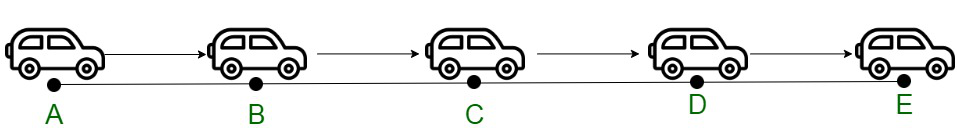Relative Motion

Relative motion can be termed as the motion of an object with respect to some other object either at rest or in motion, that is, either moving or stationary object. The motion of the object is not calculated in reference to the surface of the earth.

The assumption while computing relative motion is the understanding that the other object is in a static state. Relative motion encompasses all aspects of motion, that is, velocity, speed, or acceleration.

If we consider a person sitting in an airplane, the person appears to be at a zero velocity relative to the airplane, but actually moves with the same velocity as that of the plane taking into account the ground. In this motion, it is dependent on the observed by the observer-dependent on the observer’s frame of reference. The objects may be moving in the same or different directions with reference to each other.

Relative Velocity

The relative velocity of the body comes into consideration when the other object moves either in the same or opposite direction. The speeds of the involved objects may be increasing, decreasing, or constant with reference to each other.

Let us assume the initial position of two objects A and B are both at origin, at points xA (0) and xB (0) respectively. The corresponding positions of these objects at time instance t will be equivalent to,

xA (t) = xA (0) + vA t

xB (t) = xB (0) + vB t

Displacement from object A to B is given by,

[xB (0) – xA (0)] + (vB -vA)t

The velocity of B relative to A is given by,

vBA = vB – vA

The velocity of A relative to B is given by,

vAB = vA – vB

P-T Graphs Depicting Relative Velocities

• Two objects moving in the same direction with equal velocities.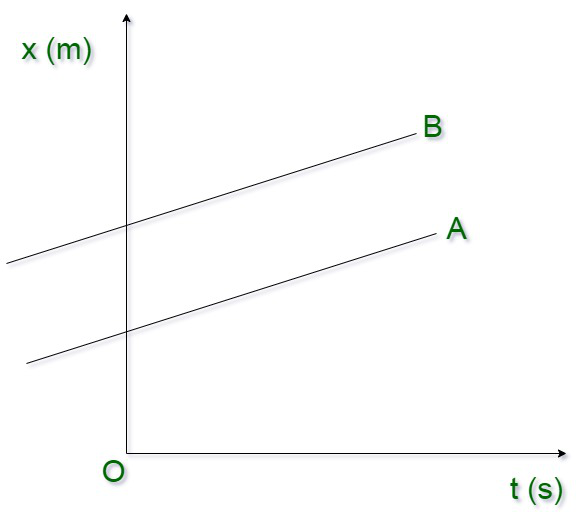VB = VA, VBA = VAB = 0

• Two objects visibly stationary for one another.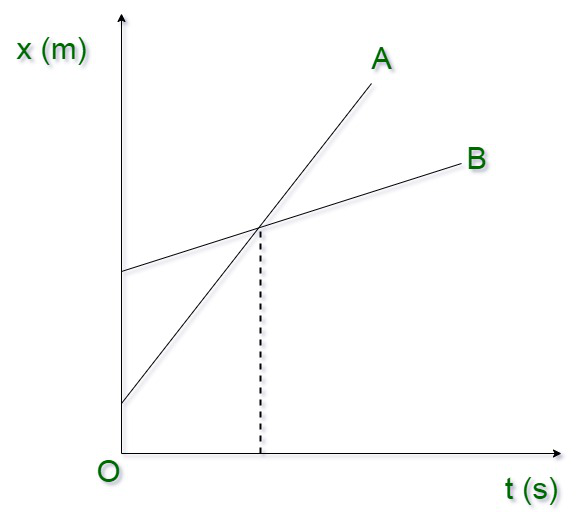VA > VB, VBA = -VAB

• The magnitude of VBA and VAB will be lower than the magnitude of VA and VB. Object A appears faster than B.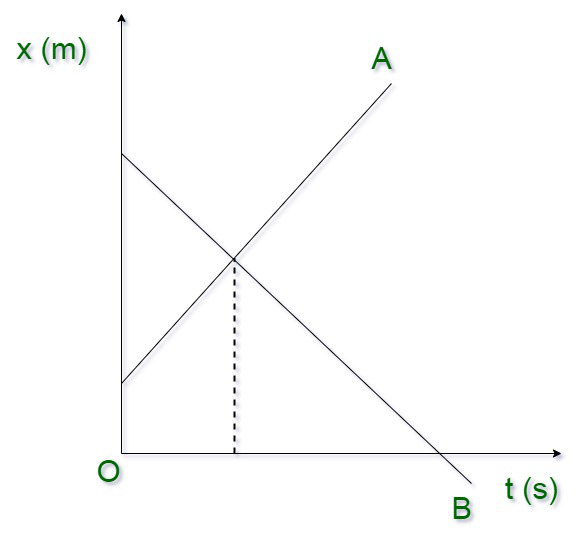VA and VB of opposite sign: VBA = -VAB

• The magnitude of VBA and VAB will be higher than the magnitude of VA and VB. Both objects will appear moving faster to one another.

Reference Frames

The velocity of an object is always considered with the reference to a time and reference (position) frame. The object takes a uniform velocity with a particularly visible frame of reference. The frame of reference is considered to be the surface of the earth for all practical purposes.

For instance, in consideration of the movement of the objects, then the surface of the earth is assumed to be the frame of reference and all the motion is considered with reference to this static point. The objects may be either in the same or opposite direction. Relative motion can be considered either along a straight line or in a plane, in multiple dimensions.

Relative Motion in One Dimension

The velocity of an object can be considered in either of the two directions, same or opposite.  The following cases may arise :

• Objects may be moving in the same direction with reference to each other.

If the object and the person are moving in the same direction, for instance, a train and a person with the surface of Earth as the reference frame. The velocity of the train with respect to the Earth can be written as:This speed can be considered to be positive.

• Objects may be moving in opposite directions with reference to each other.

If the object and the person are moving in the same direction, for instance, a train and a person moving backward towards the station with the surface of Earth as the reference frame. The velocity of a train with respect to the Earth can be written as;This speed can be considered to be negative.

The summation of the velocity vectors can be computed to find the velocity of the person with respect to Earth. The relative velocity is given by,The ordering of subscripts can be arranged to suit the requirements. During the construction of the vector equation, the subscripts for the coupling reference frame appear consecutively towards the inner reference frame.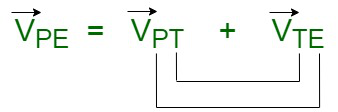Figure 1

Let us assume the first velocity of the objects moving in the same direction to be 10 m/s. In the second case, the velocity of the person moving in the opposite direction to the train, to be -2 m/s. Adding the vectors, we find,leads to the conclusion that the person is moving 8 m/s east with respect to Earth. Velocity vectors of the train with respect to Earth, person with respect to the train, and person with respect to Earth.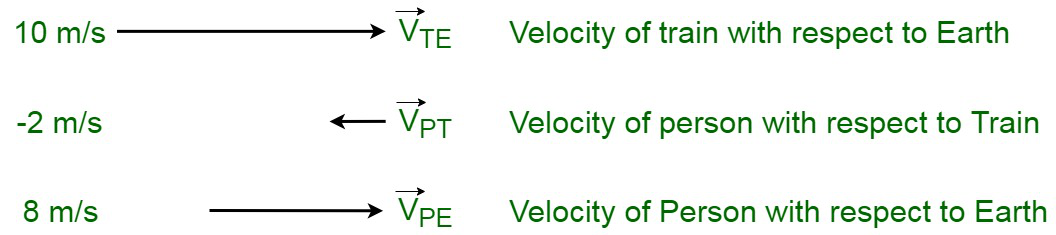Figure 2

Relative Motion in Two Dimensions

Let us consider two objects, P and Q, each of them traveling with uniform velocities, v1 and v2 respectively. The movement of the objects is considered to be parallel to each other in the same direction. The starting time instance was 0, at the origin. Let us assume their displacements from the origin X01 and X02 at some other time instance.

At time ‘t’: The displacements become x1 and x2 with respect to the origin with the position axis, the position of object P at a particular time instance becomes:

x1 = x01 + v1t    …….(1)

Similarly, for object Q :

x2 = x02 + v2t   …….(2)

Equating the equations.

Subtracting equation (1) from (2), we get:

(x2 – x1) = (x02 – x01) + (v2 – v1)t  …….(3)

We have, x01 and x02 are the initial displacements of the object Q with respect to the object P at the time instance ‘t = 0’, therefore the equation gets reduced to:

x0 = x02 – x01   ……(4)

On substituting the value of equation (4) in (3), we obtain:

(x2 – x1) = x0 + (v2 -v1)t  …….(5)

If we equate the equations, (x2 – x1) is the relative displacement of the object Q in reference to the positional coordinates of the object P, at the time instance of t seconds. Using this, we obtain,

x = x0 + (vQ – vP)t  …….(6)  [ Re-writing the eq. (5) ]

Also,……(7)

Thus, we obtain that the velocity is the rate of change of displacement.

• Relative Velocity for Objects Moving in the Same Direction

Using (7) , deriving for the relative velocity of the object Q with respect to the object P , while moving in the same direction.

vQP = v2 – v1   …..(8)

• Relative Velocity for Objects Moving in the Opposite Direction

Using (7), deriving for the relative velocity of the object Q with respect to the object P, while moving in the opposite direction.

vQP = v2 + v1   ……(9)

The dimension of relative velocity is the same as that of the velocity, which is given by [M0L1T-1].

Sample Problems

Problem 1: Athletes are participating in a relay race on the track, running with respective velocities ofand. Compute the relative velocity.

Solution:Now, if she moves in the opposite direction, then the equation will be:Problem 2: Consider a satellite moving along the equatorial plane with a velocity of s m/s. Find the relative velocity of the corresponding satellite with respect to the reference point to be the surface of the earth?

Solution:Consider the satellite to be moving in the direction of the rotation of the earth on its axis, therefore, the velocity becomes:Problem 3: The elevator is moving in an upward direction with a uniform acceleration ‘a’ m/s2. The man throws a rubber ball in the upward direction with a velocity v relative to the lift. The man catches the ball after a time instance of t seconds. Show that a + g = 2v/t.

Solution:

The lift frame can be assumed to be the point of reference. Therefore, the aspects of motion, acceleration, displacement, and velocity will be considered from the point of reference. When the ball returns to the man, therefore, the displacement from the lift frame becomes zero. Let us assume the velocity of the object with respect to the lift frame is v.

g – (-a) = a + g (↓)   downwards

Now,⇒or

a + g = 2(v/t) .

Problem 4: The two trains move with different velocities, t1 with 10 m/s and t2 with 15 m/s on parallel tracks in reference to each other. Compute the relative velocity of train t1 with respect to t2.

Solution:

Given,

v1 = 10 ms-1 , v2 = 15 ms-1

Relative velocity of slow train w.r.t. the fast train = v1 – v2 = 10 – 15 = – 5 ms-1

Negative sign shows that slow train appears to move westward w.r.t. fast train with a velocity of 5 ms-1.

Problem 5: Cart A is moving with a velocity of 40 ms-1 from North to South along one track, while cart B is moving in an opposite direction to the previous card with a speed of 30 ms-1 from South to North. Calculate the relative velocities of both the carts with each other.

Solution:

Let us consider the direction from North to South to be positive.

Therefore,

vA = +40 ms-1 and vB = -30 ms-1

(i) Relative velocity of B w.r.t. A = vB – vA = -30 – 40 = – 70 ms-1

Therefore, cart B appears to move from South to North with a speed of 70 ms-1 for any person sitting in cart A.

(ii) Velocity of the ground, vB = 0, since it is a stationary object.

Relative velocity of ground w.rt. A = vB – vA = 0 – 40 = – 40 ms-1

Therefore, the ground appears to move from the south to north direction with a speed of 40 ms-1 w.r.t. cart A.

My Personal Notes arrow_drop_up
Recommended Articles
Page :